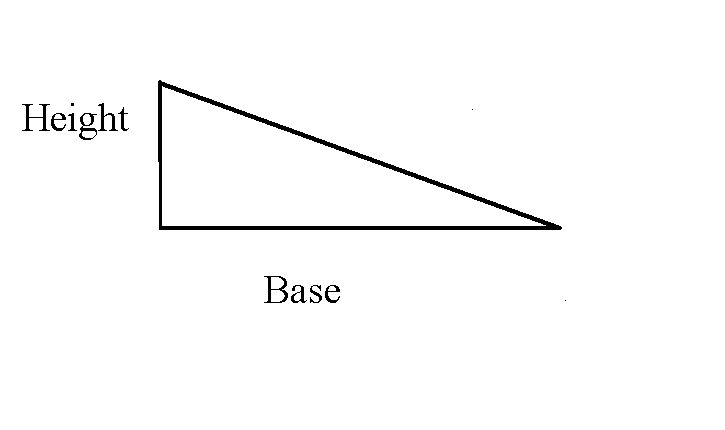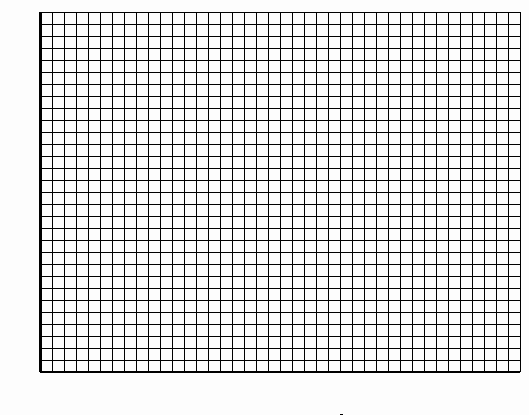PRINT THIS ANSWER SHEET TO FILL OUT AND TURN IN.

NAME ______________________________ CLASS _______________DATE ___________

FoilSim

Characteristics of Wing and Lift Area

Worksheet

1. Use FoilSim to answer the following questions:

a.) You are flying a 10,000 pound airplane (this includes personnel and cargo) at an altitude of 2,000 feet. The wings have an angle of attack of 5 degrees, a camber of 8.75%, a thickness of 15%, and an area of 100 square feet. What airspeed should you attain to have to maintain your altitude?
 WEIGHT ALTITUDE ANGLE CAMBER THICKNESS WING AREA AIRSPEED* _________ _________ _________ _________ __________ __________ _________
b.) Your plane drops 1,500 pounds of supplies to relief workers. Enter your new weight. What angle of attack will maintain your altitude of 2,000 feet?
 WEIGHT ALTITUDE ANGLE* CAMBER THICKNESS WING AREA AIRSPEED ________ __________ ________ _________ ________ ________ __________
c.) You make another drop of supplies, this time 750 pounds of food. To what value should you change your wing area to maintain your altitude of 2,000 feet?
 WEIGHT ALTITUDE ANGLE CAMBER THICKNESS WING AREA* AIRSPEED __________ __________ __________ __________ __________ __________ __________

2. The wings of the airplane you are flying are shaped like the right triangle below.a.) The total area for both of your wings is your answer in 1c. Knowing the area of a triangle = 1/2 base x height. What is the length of the base if the height of the wing is 5 feet?

Length of base =______________

b.) Suppose the total area is 120 square feet, and the base of 1 wing is 20 feet. What is the height?

Height =_____________________

3. Set the input panel to these initial conditions:

Airspeed: 100 mph

Altitude: 20,000 feet

Angle: 1.5 degrees

Thickness: 5%

Camber: 3.75%

Select 10 different values of Area between 200 and 700 square feet and record the corresponding values of Lift.

 Area (sq.ft.) Lift (lb.) 200 ________ ________ ________ ________ ________ ________ ________ ________ ________ ________ ________ ________ ________ ________ ________ ________ ________ 700 ________
a.) On the graph shown below, graph Lift vs. Area with the values you obtained.b.) What did you notice about the line connecting the points you graphed?

c.) From your graph determine the amount of lift you would generate if you had an area of 550 square feet. Check your answer by entering 550 square feet for the area and observing the amount of lift generated.

Amount of lift generated by a 550 square foot area =_____________